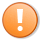# Tutorial 108 | Plotting lines at user defined times and their associated prices

## Plot lines at day open, high/low of day, high/low of user defined time period, and two user specified times

Tutorial 108 is a TradeStation EasyLanguage programming tutorial that demonstrates how to create an EasyLanguage indicator to plot lines at specific levels. The tutorial covers some of the same ground as Tutorial 45 | Finding the highest high for a period specified by start and end times but goes into more detail.

Tutorial 108 plots at the following levels:

• Plot 1: The opening price of the day
• Plot 2:  The opening price of the bar that occurs at a user input time (Time1). Should there be no bar for this specific time, the open of the following bar is used
• Plot 3: The opening price of the bar that occurs at a user input time (Time2). Should there be no bar for this specific time, the open of the following bar is used
• Plot 4: The highest price of yesterday
• Plot 5: The lowest price of yesterday
• Plot 6: The highest price in a user specified time period
• Plot 7: The lowest price in a user specified time period

The color and shading of the plot line can be set in indicator properties.

The following video explains the development of the indicator:

### Tutorial 108 video | part 2

This program is available as a free download for Gold Pass members, who will also receive a more advanced program that draws lines at both high and low pivots. I am also making the two programs available for download.

### Tutorial 108 inputs

ONightStart( 1700 ), // The start time of a user defined session
ONightEnd( 0600 ), // The end time of a user defined session
Time1( 0900 ), // A specific user defined time that a line is drawn at its open
Time2( 1400 ); // A specific user defined time that a line is drawn at its open

### Programming issues

Tutorial 108 is a fairly easy program to understand, however, there are a couple of areas that may need further explanation.

1. The user of the program can specify the start time and end time of a user specified session. When the start time is less than the end time, checking whether or not the current time is within the session is easy. We might use:
InSession =  T > Onightstart and T < Onightend;

However if the start time is a greater number than the end time (i.e. the end time occurs the next day) the above code will not work. Instead, we can test if the start time is numerically greater then the end time and then use the following:

InSession = ( T > Onightend and T < Onightstart ) = FALSE

which basically looks for times when we are NOT in the user defined session and checks that this is FALSE.

2. The user can specify two times and the program will plot lines at the level of the opening price for that time. This can be achieved by
If T = Time1 then Price1 = O;
Plot1( Price1 ):

But what if there is no bar for a particular time? If that happened the variable Price1 would not be updated correctly and we might get a spurious result.
However we can perform a test to check if Price1 has been updated each day when the bar time is equal or greater then the user input time. This can be achieved by using:

If T >= Time1 and Price1Found = False then
Begin
Price1Found = TRUE;
Price1 = O;
End;

### Gold pass areaThis content is for members only.